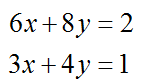Home > Grade 8 > Expressions & Equations > System of Equations, Special Case Infinitely Many Solutions

# System of Equations, Special Case Infinitely Many Solutions

Directions: Using the digits 1 to 9 at most one time each, fill in the boxes so that there are infinitely many solutions to the system of equations.### Hint

What makes a solution have infinitely many solutions?  How can you use that to start filling in the boxes?  Are there multiple possibilities?

### Answer

Here is one of many possibilities:Both linear equations must be multiples of one another.

Source: Nanette Johnson

## Square Root Expression

Directions: Use the digits 1 to 9, at most one time each, to fill in …